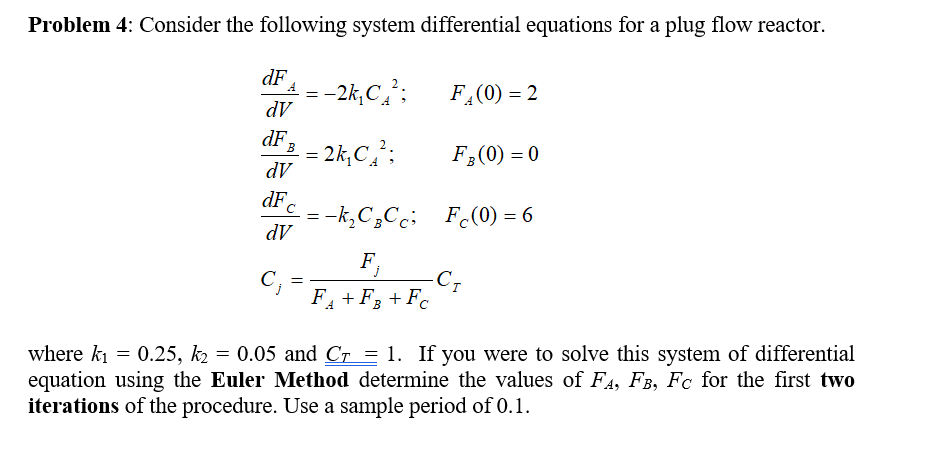# Problem 4: Consider the following system differential equations for a plug flow reactor. dF dV dF dV dF dV F (0)0 where kl = 0.25,后= 0.05 and Cr = 1. If you were to solve this system of differential equation using the Euler Method determine the values of FA, FB, Fc for the first two iterations of the procedure. Use a sample period of 0.1.

Questionhelp_outlineImage TranscriptioncloseProblem 4: Consider the following system differential equations for a plug flow reactor. dF dV dF dV dF dV F (0)0 where kl = 0.25,后= 0.05 and Cr = 1. If you were to solve this system of differential equation using the Euler Method determine the values of FA, FB, Fc for the first two iterations of the procedure. Use a sample period of 0.1. fullscreen

## Expert Answer

### Want to see this answer and more?

Experts are waiting 24/7 to provide step-by-step solutions in as fast as 30 minutes!*

*Response times vary by subject and question complexity. Median response time is 34 minutes and may be longer for new subjects.
Tagged in© 2021 bartleby. All Rights Reserved.